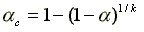# Critical Mean Difference

Given the comparison of multiple groups, the Critical Mean Difference (CMD) is the minimum difference between any two of these group means that are significant at the given level of alpha. Four methods are available: Tukey, Bonferroni, Scheffe or unprotected t-tests.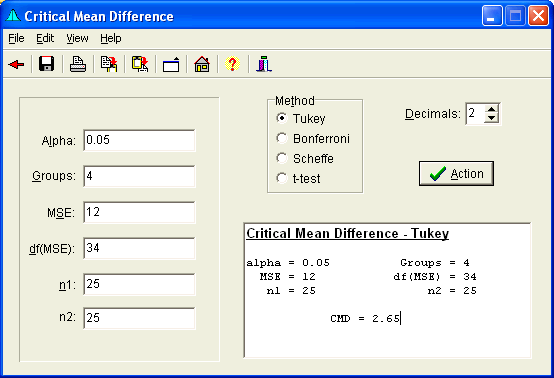## Calculations

### Tukey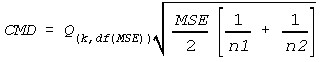### Bonnferroni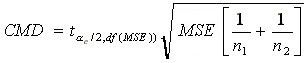### Scheffe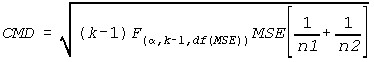### t-test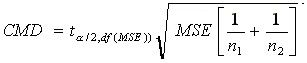Where:

• k is the number of Groups or Tests
• MSE is the Mean Square Error term
• n1 is the first group sample size
• n2 is the second group sample size
• Q is the Studentized Range Statistic
•